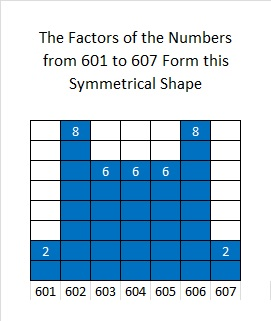# 601 The Other Side of a Twin Prime

601 and 599 are twin primes because there is only one number between them. That number happens to be 600, a number with 24 factors.

Twin primes certainly are not identical twins. That solitary number between them and the fact that they are both primes is usually all they have in common.

Other famous twin primes are 11 and 13, 17 and 19, 29 and 31, and 41 and 43.

3, 5, 7 is the only prime triplet that consists of three consecutive odd numbers because 3 is the only prime number divisible by 3, and all sets of three consecutive odd numbers must have at least one number in the set that is divisible by 3. Other than 2, 3, 5, all other so-called prime triplets come from four consecutive odd numbers. Three of the four numbers will be prime while either the second or the third number will not be.

Twin primes like 599 and 601 can be prime factors. That is something 600 can never do.

For example, 599 x 601 = 359, 999 which is 600² – 1. They are its prime factors. 600 is a factor of an infinite amount of numbers, but it is not a prime factor of any number.

What are some ways twin primes are different from each other? I’ll use twin primes 599 and 601 as examples.

When each prime in a twin prime is divided by 4, the remainder will be 1 for one of them and 3 for the other. The one that has a remainder of 1 will be the sum of two square numbers and will also be the hypotenuse of a primitive Pythagorean triple. The one with a remainder of 3 will never be either of those things. In the triple and twin primes listed above 5, 13, 17, 29, 41, and 601 have a remainder of 1 when divided by 4.

601 = 24² + 5², and is the hypotenuse of the primitive Pythagorean triple 480, 551, 601 while 599 doesn’t do either of those things.

599 is the smallest number whose digits add up to 23. If you add up 601’s digits, you’ll get 7. Figure out how many numbers less than 601 have digits that add up to 7. It’s a lot.

601 has accomplished some other impressive things:1 + 5 + 10 + 15 + 20 + 25 + 30 + 35 + 40 + 45 + 50 + 55 + 60 + 65 + 70 + 75 = 601 which makes 601 the 16th centered pentagonal number

Because it is a centered pentagonal number, 601 is one more than five times the 15th triangular number.

The factors of numbers from 601 to 607 form a symmetrical shape.I gave the factoring information for 599 previously. Here is the information for 601:

• 601 is a prime number.
• Prime factorization: 601 is prime.
• The exponent of prime number 601 is 1. Adding 1 to that exponent we get (1 + 1) = 2. Therefore 601 has exactly 2 factors.
• Factors of 601: 1, 601
• Factor pairs: 601 = 1 x 601
• 601 has no square factors that allow its square root to be simplified. √601 ≈ 24.5153How do we know that 601 is a prime number? If 601 were not a prime number, then it would be divisible by at least one prime number less than or equal to √601 ≈ 24.5. Since 601 cannot be divided evenly by 2, 3, 5, 7, 11, 13, 17, 19, or 23, we know that 601 is a prime number.